Next: Implementation and Performance Up: Finding Good Matchings Previous: Matching Criteria for

## A Matching Algorithm

Theorem 5.1 allows us to design a simple algorithm for computing the best matching. This algorithm, called algorithm Match, is presented in [CRGMW95], and runs in quadratic time. Below, we present a faster algorithm, called FastMatch, for computing the unique maximal matching. Our algorithm uses a function equal to compare nodes. For leaf nodes,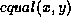is true if and only if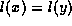and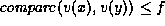, where f is a parameter valued between 0 and 1. For internal nodes,is true if and only ifand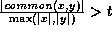, where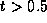is a parameter.

Figure 10 shows Algorithm FastMatch, which uses the longest common subsequence (LCS) routine, introduced earlier in Section 4.2, to perform an initial matching of nodes that appear in the same order. Nodes still unmatched after the call to LCS are processed using linear search. We assume that all nodes with a given label l in tree T are chained together from left to right. Let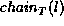denote the chain of nodes with label l in tree T. Node x occurs to the left of node y inif x appears before y in the in-order traversal of T when siblings are visited left-to-right.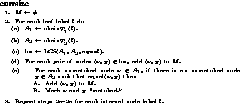Figure 10: Algorithm FastMatch

Now we analyze the running time of Algorithm FastMatch. Define the weighted edit distance e between trees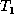and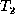as follows. Let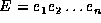be the shortest edit script that transformsto. Then the weighted edit distance is given by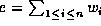where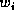, for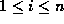, is 1 if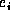is an insert or a delete, |x| ifis a move of the subtree rooted at x, and 0 otherwise. Recall that |x| denotes the number of leaf nodes that are descendants of node x. Intuitively, the weighted edit distance indicates how different the two trees are ``structurally,'' where the degree of difference associated with the move of a subtree depends on the number of leaves in the subtree. In [CRGMW95] we show that the running time of Algorithm FastMatch is proportional to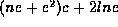where n is the total number of leaf nodes, c is the average cost of comparing two leaves (using the compare function), l is the number of internal node labels, and e is the weighted edit distance betweenand.Next: Implementation and Performance Up: Finding Good Matchings Previous: Matching Criteria for

Sudarshan S. Chawathe
Wed Jun 19 08:22:34 PDT 1996